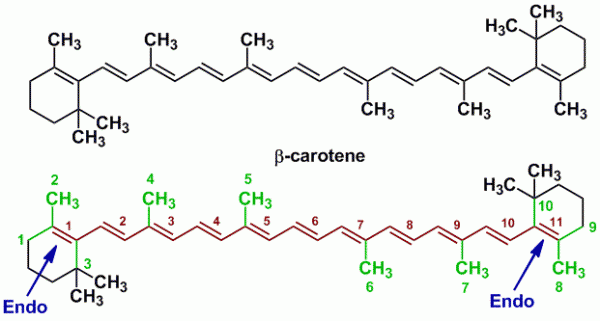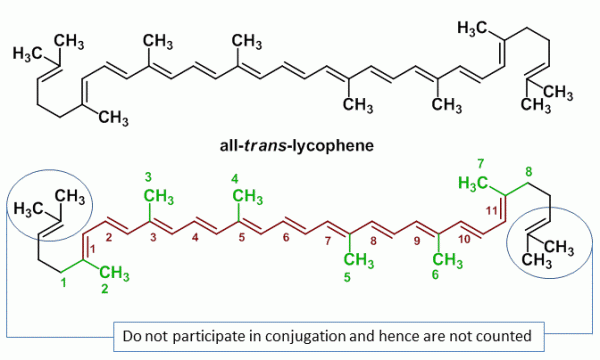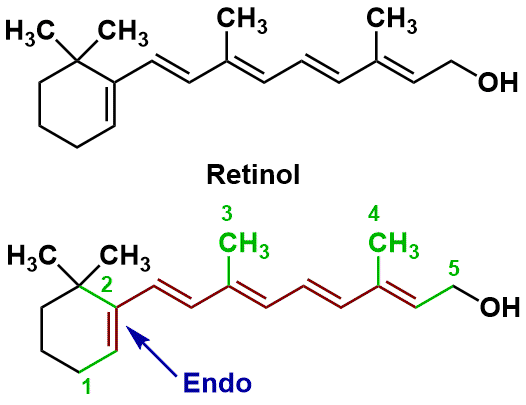# Ultraviolet-Visible (UV-Vis) Spectroscopy – Fieser-Kuhn Rules to Calculate Wavelength of Maximum Absorption (Lambda-max) of Polyenes (with Sample Problems)

## Introduction

Woodward-Fieser rules work well for conjugated dienes and polyenes with upto 4-double bonds or less. Certain plant pigments such as carotenoids have even more than 4 conjugated double bonds. For conjugated polyenes having more than 4 double bonds, the Fieser-Kuhn rules must be applied in order to obtain the wavelength of maximum absorption.

## Fieser-Kuhn Rule for Conjugated Polyenes

According to the Fieser-Kuhn rule the following equation can be used to solve for the wavelength of maximum absorption λmax and also maximum absorptivity εmax:

λmax = 114 + 5M + n (48.0 – 1.7 n) – 16.5 Rendo – 10 Rexo

where,
λmax is the wavelength of maximum absorption
M is the number of alkyl substituents / ring residues in the conjugated system
n is the number of conjugated double bonds
Rendo is the number of rings with endocyclic double bonds in the conjugated system
Rexo is the number of rings with exocyclic double bonds in the conjugated system.

and

εmax = (1.74 x 104) n

where,
εmax
is the maximum absorptivity
n is the number of conjugated double bonds.

Thus using the above equations, one can get the wavelength of maximum absorbance (λmax) and the maximum absorptivity (εmax)

## Sample Problem 1: β-Carotene

β-carotene is a precursor of vitamin A which is a terpenoid derived from several isoprene units. The observed λmax of β-carotene is 452 nm, while the observed εmax is 15.2 x 104. Let us therefore use Fieser-Kuhn rules to calculate the λmax and the εmax for β-carotene.Name of Compound β-Carotene Base Value 114 nm M (number of alkyl substituents) 10 n (number of conjugated double bonds) 11 Rendo (number of endocyclic double bonds) 2 Rexo (number of exocyclic double bonds) 0 Substituting in equation λmax = 114 + 5M + n (48.0 – 1.7 n) – 16.5 Rendo – 10 Rexo = 114 + 5(10) + 11 (48.0-1.7(11)) – 16.5 (2) – 10 (0)= 114 + 50 + 11 (29.3) – 33 – 0= 114 + 50 + 322.3 – 33 Calc. λmax = 453.30 nm λmax observed practically 452nm Calculate εmax using equation: εmax = (1.74 x 104) n = (1.74 x 104) 11Calc. εmax= 19.14 x 104 Practically observed εmax 15.2 x 104

## Sample Problem 2: all-trans-Lycophene

Lycophene (all-trans-lycophene) is a bright red carotenoid pigment found in tomatoes and other red fruits and vegetables. However, lycophene has no vitamin A like activity.Name of Compound all-trans-lycophene Base Value 114 nm M (number of alkyl substituents) 8 n (number of conjugated double bonds) 11 Rendo (number of endocyclic double bonds) 0 Rexo (number of exocyclic double bonds) 0 Substituting in equation λmax = 114 + 5M + n (48.0 – 1.7 n) – 16.5 Rendo – 10 Rexo = 114 + 5(8) + 11 (48.0-1.7(11)) – 16.5 (0) – 10 (0)= 114 + 40 + 11 (29.3) – 0 – 0= 114 + 40 + 322.3 – 0 Calc. λmax = 476.30 nm λmax observed practically 474nm Calculate εmax using equation: εmax = (1.74 x 104) n = (1.74 x 104) 11Calc. εmax= 19.14 x 104 Practically observed εmax 18.6 x 104

## Sample Problem 3: Retinol

Retinol is an animal form of vitamin A and is essential for vision.Name of Compound Retinol Base Value 114 nm M (number of alkyl substituents) 5 n (number of conjugated double bonds) 5 Rendo (number of endocyclic double bonds) 1 Rexo (number of exocyclic double bonds) 0 Substituting in equation λmax = 114 + 5M + n (48.0 – 1.7 n) – 16.5 Rendo – 10 Rexo = 114 + 5(5) + 5 (48.0-1.7(5)) – 16.5 (1) – 10 (0) = 114 + 25 + 5 (39.5) – 16.5 – 0 = 114 + 25 + 197.5 – 16.5 – 0 Calc. λmax = 320 nm λmax observed practically 325 nm Calculate εmax using equation: εmax = (1.74 x 104) n = (1.74 x 104) 5Calc. εmax= 8.7 x 104 Practically observed εmax N/A

## Books on Analytical Chemistry and Spectroscopy

Check out these good books for analytical chemistry and spectroscopy

#### About The Author

1.2.3.4.5.6.7.8.9.10.11.•••12.••13.